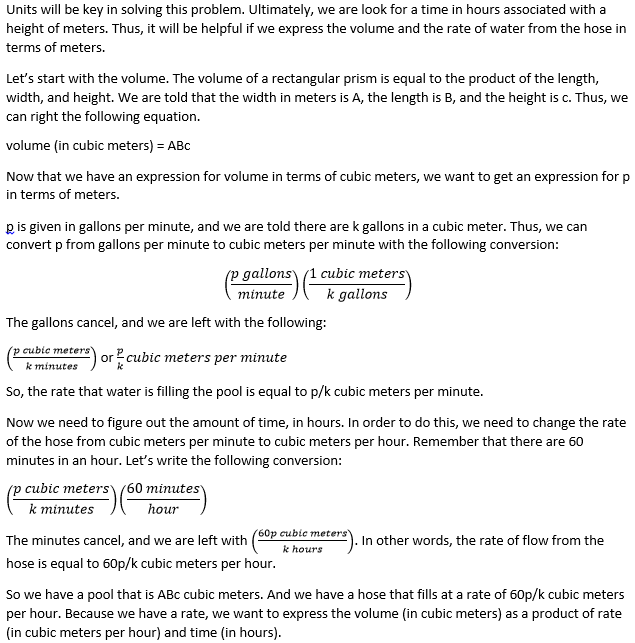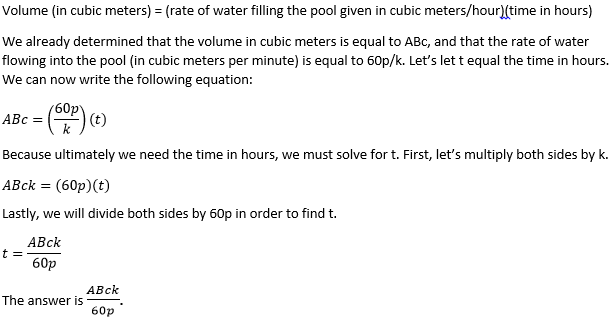# PSAT Math : Prisms

## Example Questions

← Previous 1 3

### Example Question #1 : Prisms

For a box to fit inside the cupboard, the sum of the height and the perimeter of the box must, at most, be 360 cm. If Jenn has a box that has a height of 40 cm and a length of 23 cm, what is the greatest possible width of the box?

207 cm

13 cm

0.4 cm

297 cm

137 cm

137 cm

Explanation:

First we write out the equation we are given. H + (2L +2W) = 360.  = 40 and = 23

40 + (2(23) + 2W) = 360

40 + (46 + 2W) = 360

46 + 2W = 320

2W = 274

W = 137

### Example Question #2 : Prisms

The volume of a rectangular prism is 80 cm3.  The length, width, and height of the prism are each an integer number of cm.  If the dimensions form three terms of an arithmetic sequence, find the average of the three dimensions.

5

8

6

4

7

5

Explanation:

Method 1:

Trial and error to find a combination of factors of 80 that differ by the same amount will eventually yield 2, 5, 8.  The average is 5.

Method 2:

Three terms of an arithmetic sequence can be written as x, x+d, and x+2d. Multiply these together using the distributive property to find the volume and the following equation results:

x3 + 3dx2 + 2d2x - 80 = 0

Find an integer value of x that creates an integer solution for d.  Try x=1 and we see the equation 1 + 3d + 2d2 - 80 = 0 or 2d2 + 3d -79 = 0.  The determinant of this quadratic is 641, which is not a perfect square.  Therefore, d is not an integer when x=1.

Try x=2 and we see the equation 8 + 12d + 4d2 - 80 = 0 or d2 + 3d - 18 = 0.  This is easily factored to (d+6)(d-3)=0 so d=-6 or d=3.  Since a negative value of d will result in negative dimensions of the prism, d must equal 3.  Therefore, when substituting x=2 and d=3, the dimensions x, x+d, and x+2d become 2, 5, and 8.  The average is 5.

### Example Question #3 : Prisms

A right rectangular prism has a volume of 64 cubic units. Its dimensions are such that the second dimension is twice the length of the first, and the third is one-fourth the dimension of the second. What are its exact dimensions?

3 x 6 x 12

4 x 4 x 4

1 x 2 x 32

1 x 4 x 16

2 x 4 x 8

2 x 4 x 8

Explanation:

Based on our prompt, we can say that the prism has dimensions that can be represented as:

Dim1: x

Dim2: 2 * Dim1 = 2x

Dim3: (1/4) * Dim2 = (1/4) * 2x = (1/2) * x

More directly stated, therefore, our dimensions are: x, 2x, and 0.5x. Therefore, the volume is x * 2x * 0.5x = 64, which simplifies to x3 = 64. Solving for x, we find x = 4. Therefore, our dimensions are:

x = 4

2x = 8

0.5x = 2

Or: 2 x 4 x 8

### Example Question #4 : Prisms

A right rectangular prism has a volume of 120 cubic units. Its dimensions are such that the second dimension is three times the length of the first, and the third dimension is five times the dimension of the first. What are its exact dimensions?

1 x 5 x 24

2 x 6 x 10

4 x 12 x 20

2 x 5 x 12

2 x 6 x 10

Explanation:

Based on our prompt, we can say that the prism has dimensions that can be represented as:

Dim1: x

Dim2: 3 * Dim1 = 3x

Dim3: 5 * Dim1 = 5x

More directly stated, therefore, our dimensions are: x, 3x, and 5x. Therefore, the volume is x * 3x * 5x = 120, which simplifies to 15x3 = 120 or x3 = 8. Solving for x, we find x = 2. Therefore, our dimensions are:

x = 2

3x = 6

5x = 10

Or: 2 x 6 x 10

### Example Question #1 : Volume Of A Rectangular Solid

A rectangular box has a length of 2 meters, a width of 0.5 meters, and a height of 3.2 meters. How many cubes with a volume of one cubic centimeter could fit into this rectangular box?

3.2 x 10-3

3.2

3,2 x 103

3.2 x 106

3.2 x 102

3.2 x 106

Explanation:

In order to figure out how many cubic centimeters can fit into the box, we need to figure out the volume of the box in terms of cubic centimeters. However, the measurements of the box are given in meters. Therefore, we need to convert these measurements to centimeters and then determine the volume of the box.

There are 100 centimeters in one meter. This means that in order to convert from meters to centimeters, we must multiply by 100.

The length of the box is 2 meters, which is equal to 2 x 100, or 200, centimeters.

The width of the box is 0.5(100) = 50 centimeters.

The height of the box is 3.2(100) = 320 centimeters.

Now that all of our measurements are in centimeters, we can calculate the volume of the box in cubic centimeters. Remember that the volume of a rectangular box (or prism) is equal to the product of the length, width, and height.

V = length x width x height

V = (200 cm)(50 cm)(320 cm) = 3,200,000 cm3

To rewrite this in scientific notation, we must move the decimal six places to the left.

V = 3.2 x 106 cm3

The answer is 3.2 x 106.

### Example Question #1 : How To Find The Volume Of A Prism

A rectangular prism has a length that is twice as long as its width, and a width that is twice as long as its height. If the surface area of the prism is 252 square units, what is the volume, in cubic units, of the prism?

27

432

108

216

1728

216

Explanation:

Let l be the length, w be the width, and h be the height of the prism. We are told that the length is twice the width, and that the width is twice the height. We can set up the following two equations:

l = 2w

w = 2h

Next, we are told that the surface area is equal to 252 square units. Using the formula for the surface area of the rectangular prism, we can write the following equation:

surface area = 2lw + 2lh + 2wh = 252

We now have three equations and three unknowns. In order to solve for one of the variables, let's try to write w and l in terms of h. We know that w = 2h. Because l = 2w, we can write l as follows:

l = 2w = 2(2h) = 4h

Now, let's substitute w = 2h and l = 4h into the equation we wrote for surface area.

2(4h)(2h) + 2(4h)(h) + 2(2h)(h) = 252

Simplify each term.

16h2 + 8h2 + 4h2 = 252

Combine h2 terms.

28h2 = 252

Divide both sides by 28.

h2 = 9

Take the square root of both sides.

h = 3.

This means that h = 3. Because w = 2h, the width must be 6. And because l = 2w, the length must be 12.

Because we now know the length, width, and height, we can find the volume of the prism, which is what the question ultimately requires us to find.

volume of a prism = l • w • h

volume = 12(6)(3)

= 216 cubic units

### Example Question #1950 : Hspt Mathematics

The dimensions of Treasure Chest A are 39” x 18”. The dimensions of Treasure Chest B are  16” x 45”. Both are 11” high. Which of the following statements is correct?

Treasure Chest B can hold more treasure.

There is insufficient data to make a comparison between Treasure Chest A and Treasure Chest B.

Treasure Chest A and B can hold the same amount of treasure.

Treasure Chest A has the same surface area as Treasure Chest B.

Treasure Chest A can hold more treasure.

Treasure Chest B can hold more treasure.

Explanation:

The volume of B is 7920 in3. The volume of A is 7722 in3. Treasure Chest B can hold more treasure.

### Example Question #1 : Prisms

Carlos has a pool in the shape of a rectangular prism. He fills the pool with a hose that ejects water at a rate of p gallons per minute. The bottom of the pool is A meters wide and B meters long. If there are k gallons in a cubic meter, then which of the following expressions will be equal to the amount of time it takes, in hours, for the water in the pool to reach a height of c meters?

60ABc/(pk)

60ABcp/k

ABc/(pk)

ABck/(60p)

ABcp/(60k)

ABck/(60p)

Explanation:### Example Question #1 : How To Find The Diagonal Of A Prism

The dimensions of a right, rectangular prism are 4 in x 12 in x 2 ft. What is the diagonal distance of the prism?

4√(3)

8√(7)

4√(46)

8√(23)

4√(46)

Explanation:

The problem is simple, but be carefulThe units are not equal. First convert the last dimension into inches. There are 12 inches per foot. Therefore, the prism's dimensions really are: 4 in x 12 in x 24 in.

From this point, things are relatively easy. The distance from corner to corner in a three-dimensional prism like this can be found by using a variation on the Pythagorean Theorem that merely adds one dimension. That is, d2  = x2y2 + z2, or d√(x2y2 + z2)

For our data, this would be:

d = √(42 + 122 + 242) = √(16 + 144 + 576) = √(736) = √(2 * 2 * 2 * 2 * 2 * 23) = 4√(46)

### Example Question #2 : How To Find The Diagonal Of A Prism

The base of a right, rectangular prism is a square. Its height is three times that of one of the sides of the base. If its overall volume is 375 in3, what is the diagonal distance of the prism?

25√(11) in

5 in

5√(11) in

5√(3) in

5√(11) in

Explanation:

First, let's represent our dimensions. We know the bottom could be represented as being x by x. The height is said to be three times one of these dimensions, so let's call it 3x. Based on this, we know the dimensions of the prism are x, x, and 3x. Now, the volume of a right rectangular prism is found by multiplying together its three dimensions. Therefore, if we know the overall volume is 375 in3, we can say:

375 = x * x * 3x or 375 = 3x3

Simplifying, we first divide by 3: 125 = x3. Taking the cube root of both sides, we find that x = 5.

Now, be careful. The dimensions are not 5, 5, 5. They are (recall) x, x, and 3x. If x = 5, this means the dimensions are 5, 5, and 15.

At this point, things are beginning to progress to the end of the problem. The distance from corner to corner in a three-dimensional prism like this can be found by using a variation on the Pythagorean Theorem that merely adds one dimension. That is, d2  = x2 + y2 + z2, or d = √(x2 + y2 + z2)

For our data, this would be: d = √(52 + 52 + 152) = √(25 + 25 + 225) = √(275) = √(5 * 5 * 11) = 5√(11) in

← Previous 1 3